Precalculus : Find the value of any of the six trigonometric functions

Example Questions

Example Question #1 : Trigonometric Functions

Solve the following: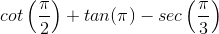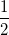Explanation:

Rewritein terms of sine and cosine functions.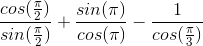Since these angles are special angles from the unit circle, the values of each term can be determined from the x and y coordinate points at the specified angle.

Solve each term and simplify the expression.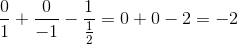Example Question #1 : Trigonometric Functions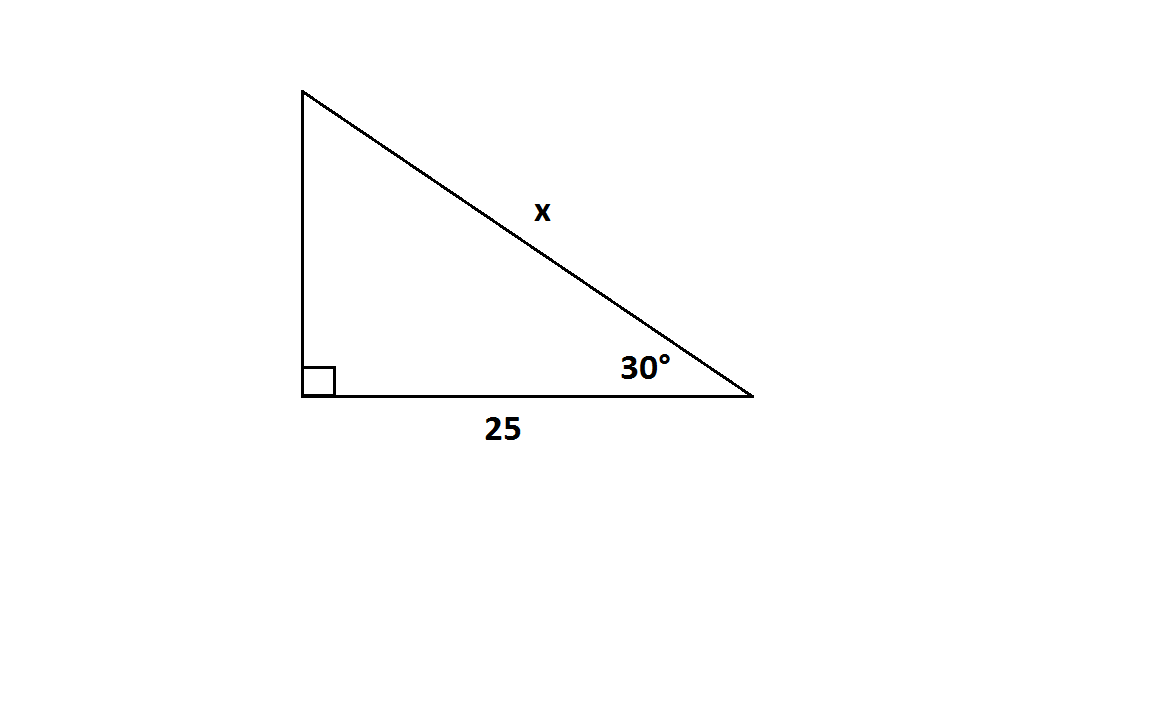Find the value of.Explanation:

Using trigonometric relationships, one can set up the equation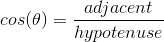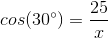.

Solving for,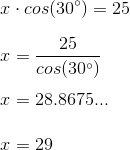Thus, the answer is found to be 29.

Example Question #1 : Trigonometric Functions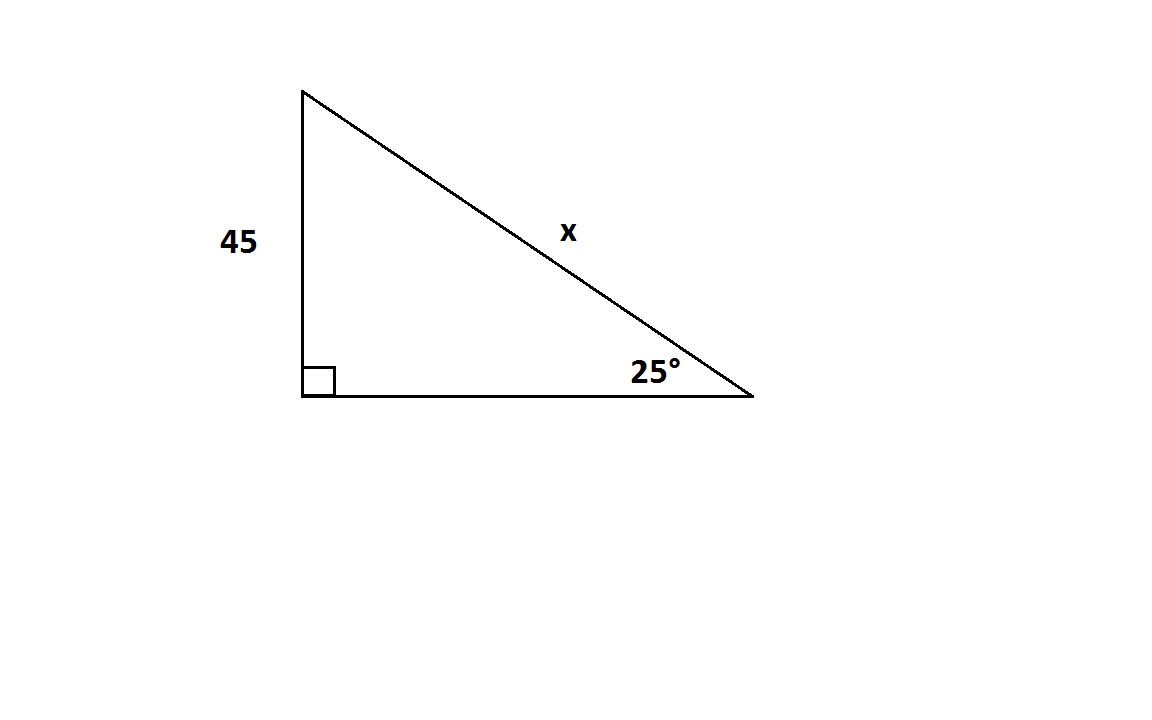Find the value of.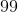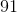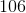Explanation:

Using trigonometric relationships, one can set up the equation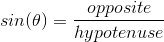.

Plugging in the values given in the picture we get the equation,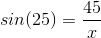.

Solving for,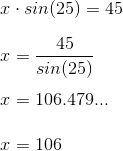.

Thus, the answer is found to be 106.

Example Question #1 : Trigonometric Functions

Find all of the angles that satistfy the following equation: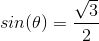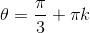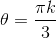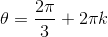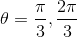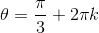ORORExplanation:

The values of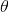that fit this equation would be: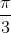and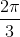because these angles are in QI and QII where sin is positive and where.is incorrect, because it includes inputs that provide negative values such as: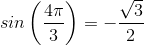Thus the answer would be eachmultiple ofand, which would provide the following equations:ORAll Precalculus Resources